# Oscillations: the Essentials

3/22/07

### Complex Numbers

Be familiar with the complex plane: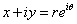, definitions: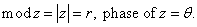To multiply complex numbers, multiply the mods, add the phases.  Know the all-important formula:and be able to interpret it as a point on the unit circle.

Some useful small x approximations: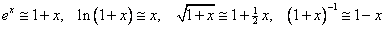and for small angles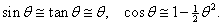### Undamped Simple Harmonic Oscillator:

Be able to solve the equation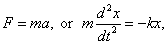and write down the velocity and kinetic energy at any time.  Be able to sketch a graph of the potential energy as a function of position, both for a horizontal and a vertical spring.  Be able to derive the angular frequency and the period.  Know how to find the dependence of the period on k, m using dimensional arguments.

### A Heavily Damped Oscillator:

You should know the equation of motion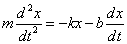and that a solution is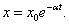Be able to substitute this in the equation to find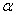and from that the conditionfor exponential decay.

Be able to use dimensional arguments to find that m/b and b/k  both have dimensions of time, and for large damping give a physical interpretation of when these very different times are physically relevant.

### A Lightly Damped Oscillator:

I would not ask you to solve the equation of motion in this case, but you should know the following facts: the energy in the oscillator decays in time aswhere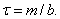The Q factor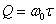measures how many radians the oscillator goes through during the time the energy drops by a factor 1/e.   You should be able to sketch how the amplitude decays in time, showing the exponential “envelope” of the oscillations.

### Critical Damping:

Know the condition for critical damping, and be able to sketch how the amplitude decays above, at and below critical damping.

### Principle of Superposition:

For the damped simple harmonic oscillator reviewed above, if f1(x) and f2(x) are solutions, so is A1f1(x) +A2f2(x) for any constants A1, A2.  However, this is not the case for the driven oscillator reviewed below: in fact, if f1(x) is a solution for the driven oscillator, 2 f1(x) would be a solution if the driving force were doubled.  But one can add to a solution of the driven oscillator any solution of the same but undriven oscillator—and this is usually necessary to fit initial conditions.

### A Driven Damped Oscillator:

Be able to write down the equation of motion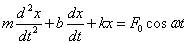and understand it as the real part of the equationKnow how the put in the trial solution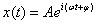and from that deduce the amplitude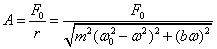.  Be familiar with the form of this as a function of driving frequency, especially near resonance.

### The Pendulum:

Know the equation of motion of a simple pendulum, how it relates to a simple harmonic oscillator, how to handle the equation if the pendulum is a rigid body rather than just a point mass on a light rod or string.  Know how to find the potential energy as a function of angle.